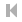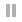# Graphtoy v0.4

by Inigo Quilez (feedback from Rafæl Couto, Florian Mosleh, Nicholas Ralabate and Rich Eakin). If you find Graphtoy useful, please consider supporting it by donating through my Patreon or PayPal.
Dark
Grid Dec
Free
(0.0, 0.0)
t = 0.0Clear
Ex 1Example 1
Ex 2Example 2
Ex 2Example 3
f1(x,t) =
f2(x,t) =
f3(x,t) =
f4(x,t) =
f5(x,t) =
f6(x,t) =

()
+
-
*
/
rcp(x)
fma(x,y,z)
%
mod(x,y)
^
**
pow(x,y)
exp(x)
exp2(x)
exp10(x)
log(x)
log2(x)
log10(x)
sqrt(x)
cbrt(x)
rsqrt(x)
rcbrt(x)
inversesqrt(x)
abs(x)
sign(x)
ssign(x)
cos(x)
sin(x)
tan(x)
acos(x)
asin(x)
atan(x)
atan2(x,y)
degrees(x)
cosh(x)
sinh(x)
tanh(x)
acosh(x)
asinh(x)
atanh(x)
ceil(x)
floor(x)
trunc(x)
round(x)
frac(x)
fract(x)
min(x,y)
max(x,y)
saturate(x)
clamp(x,c,d)
step(a,x)
smoothstep(a,b,x)
over(x,y)
remap(a,b,x,c,d)
mix(a,b,x)
lerp(a,b,x)
tri(a,x)
sqr(a,x)
noise(x)
cellnoise(x)
voronoi(x)
PI
E
PHI
LN10
LN2
LOG10E
LOG2E
SQRT2
SQRT1_2
𝜋
𝜏
φ
²
³
½
¼
¾

• Use Mouse to pan view
• Use SHIFT+Mouse to zoom centered at the mouse pointer
• Use Mouse Wheel to zoom on current viewport center